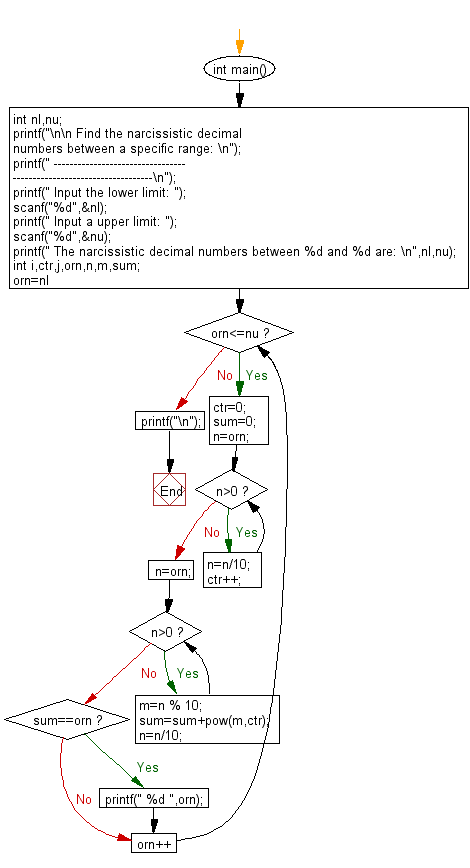﻿ C Program: Narcissistic decimal numbers within a range# C Exercises: Find narcissistic decimal numbers within a specific range

## C Numbers: Exercise-34 with Solution

Write a program in C to find narcissistic decimal numbers within a specific range.

Test Data
Input the lower limit: 100
Input a upper limit: 1000

Sample Solution:

C Code:

``````# include <stdio.h>
# include <stdlib.h>
# include <stdbool.h>
# include <math.h>

int main()
{
int nl,nu;
printf("\n\n Find the narcissistic decimal numbers between a specific range: \n");
printf(" --------------------------------------------------------------------\n");
printf(" Input the lower limit: ");
scanf("%d",&nl);
printf(" Input a upper limit: ");
scanf("%d",&nu);
printf(" The narcissistic decimal numbers between %d and %d are: \n",nl,nu);
int i,ctr,j,orn,n,m,sum;
for(orn=nl;orn<=nu;orn++)
{
ctr=0;
sum=0;
n=orn;
while(n>0)
{
n=n/10;
ctr++;
}
n=orn;
while(n>0)
{
m=n % 10;
sum=sum+pow(m,ctr);
n=n/10;
}
if(sum==orn)
{
printf(" %d ",orn);
}
}
printf("\n");
}
```
```

Sample Output:

``` Input the lower limit: 100
Input a upper limit: 1000
The narcissistic decimal numbers between 100 and 1000 are:
153  370  371  407
```

Pictorial Presentation:Flowchart:C Programming Code Editor:

What is the difficulty level of this exercise?

Test your Programming skills with w3resource's quiz.

﻿

## C Programming: Tips of the Day

Why do C and C++ compilers allow array lengths in function signatures when they're never enforced?

It is a quirk of the syntax for passing arrays to functions.

Actually it is not possible to pass an array in C. If you write syntax that looks like it should pass the array, what actually happens is that a pointer to the first element of the array is passed instead.

Since the pointer does not include any length information, the contents of your [] in the function formal parameter list are actually ignored.

Ref : https://bit.ly/3fhlvdH